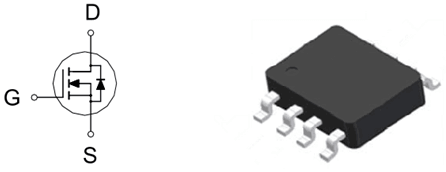# 基本元器件 - 场效应管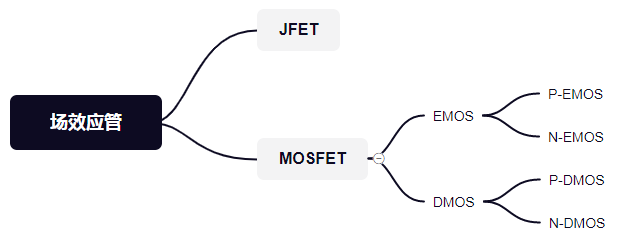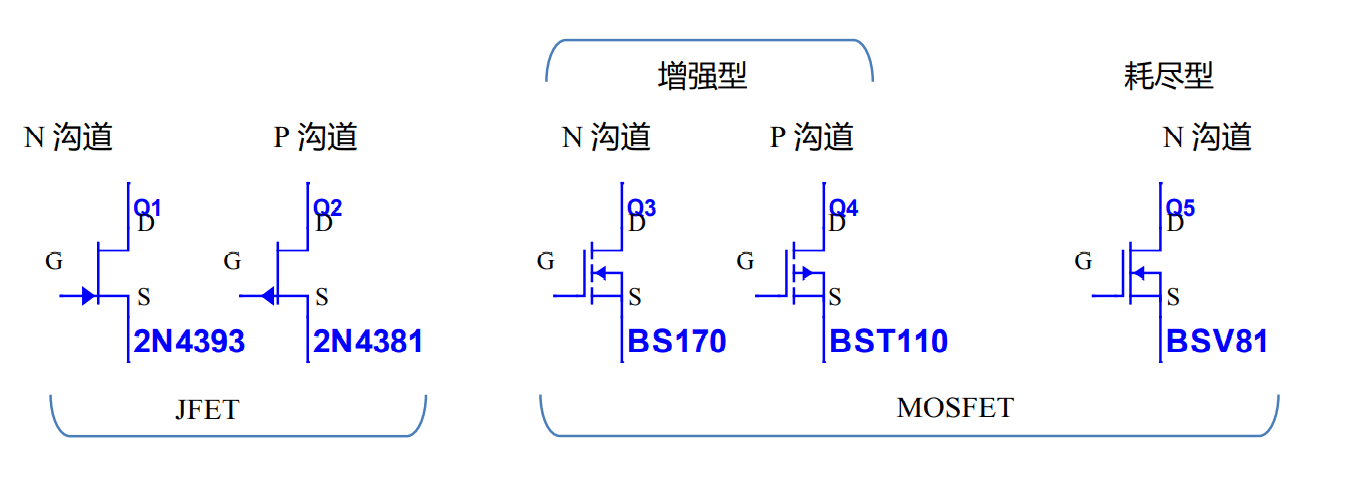## MOS 管的引脚定义

MOS 管有三个引脚（G，S，D）其定义如下：

• G：gate / 栅极
• S：source / 源极
• D：drain / 漏极

N 沟道的电源一般接在 D，输出接 S；P 沟道的电源一般接在 S，输出接 D，增强型 / 耗尽型接法基本一样。

MOS 管的 source 和 drain 是可以对调的，他们都是在 P 型 backgate 中形成的 N 型区，在大多数情况下，这个两个区是一样的，即使对调也不会影响性能。

## 寄生二极管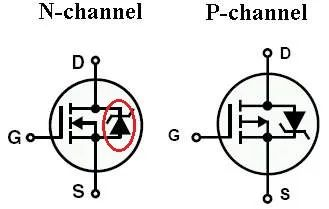## MOS 管的导通条件

MOS 管是压控型，由 G 和 S 极之间压差决定是否导通。

## 增强型 MOS 管的特性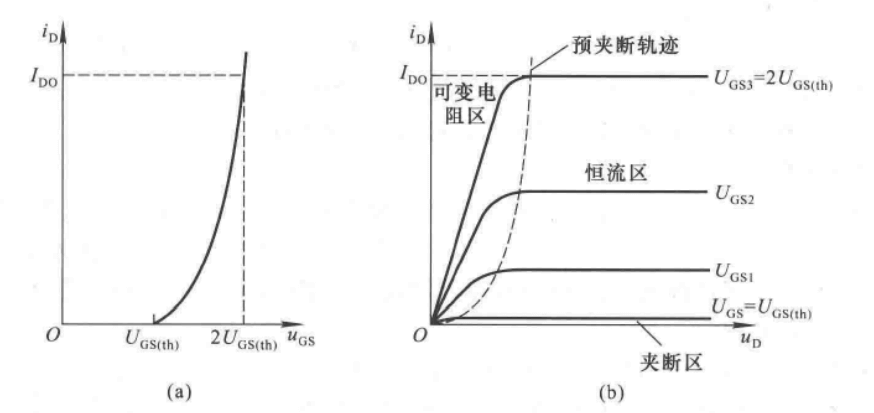1. 开启电压 $$U_{GS_(th)}$$：从图中可以看出 $$U_{GS_(th)} = 1 V$$。当 $$U_{GS} < U_{GS_(th)}$$ 时，无论 $$U_{DS}$$ 多大，电流 $$i_D$$ 始终为 0。当 $$U_{GS} > U_{GS_(th)}$$ 时，MOSFET 才算开启。
2. 恒流区方程$$i_D = K(u_{GS}-U_{GS_(th))^2$$，其中，K 影响转移特性曲线的增长速率（单位是 $$A/V^2$$
3. 可变电阻区和恒流区的分界线：随着 $$U_{GS}$$ 增加，分界点电压 $$U_{DS_{dv}}$$ 也在增加，且满足 $$U_{DS_{dv}}=U_{GS} - U_{GS_(th)}$$

## MOSFET 工作状态

MOSFET 不同于三极管，因为某些型号封装内有并联二极管，所以其 D 和 S 极是不能反接的，且 N 管必须由 D 流向 S，P 管必须由 S 流向 D。可以用下表判断工作状态：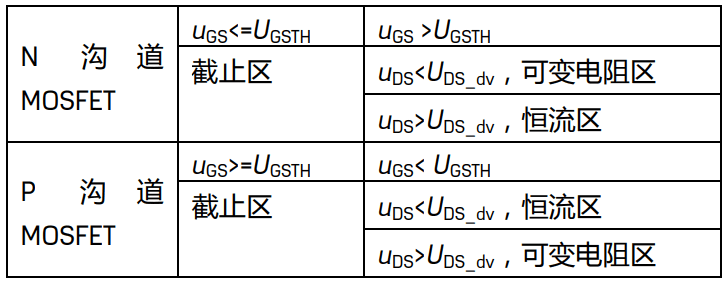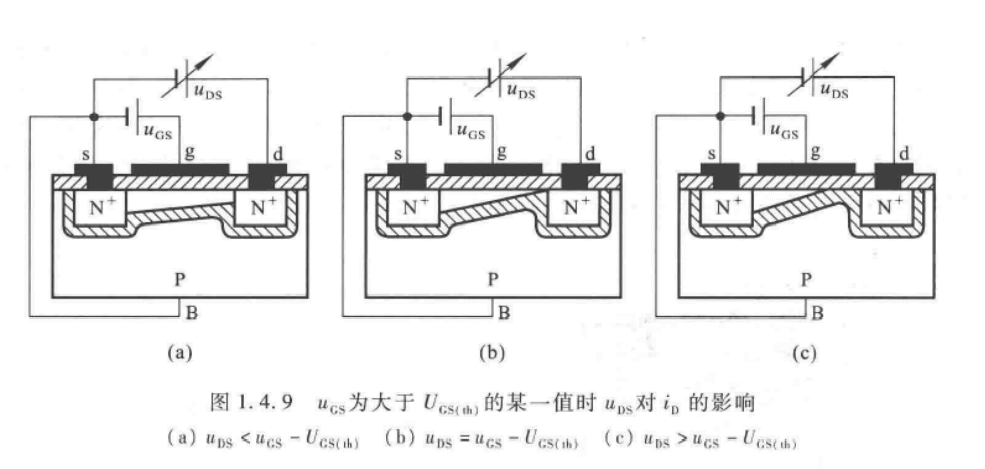• 截止区：当 $$U_{GS}$$ 小于开启电压 $$U_{GS_(th)}$$ 时，MOS 不导通。
• 可变电阻区$$U_{DS}$$ 很小，$$I_D$$$$U_{DS}$$ 增大而增大。
• 恒流区$$U_{DS}$$ 变化，$$I_D$$ 变化很小。
• 击穿区$$U_{DS}$$ 达到一定值时，MOS 被击穿，$$I_D$$ 突然增大，如果没有限流电阻，将被烧坏。
• 过损耗区：功率较大，需要加强散热，注意最大功率。

## MOSFET 主要参数

• 开启电压 $$U_{GS_(th)}$$：增强型 MOS 的参数。指当 $$U_{DS}$$ 不变时，使得 $$i_D > 0$$ 所需最小的 $$\left| u_{GS} \right|$$ 的值。
• 夹断电压 $$U_{GS_(off)}$$：结型场效应管和耗尽型 MOS 的参数，与 $$U_{GS_(th)}$$ 相似，代表当 $$U_{DS}$$ 不变时，$$i_D$$ 为规定的微小电流时的 $$u_{GS}$$
• 直流输入电阻 $$U_{GS_(DC)}$$：栅 - 源电压与栅极电流之比，一般 MOS 的 $$U_{GS_(DC)} > 10^9 \Omega$$

1. 击穿电压 V_BRDSS
• 随温度变化，应留足余量
2. 导通电阻 R_DS(on)
• 导通电阻正温度系数，适合并联工作
• 导通电阻越小，导通损耗越小
• 导通电阻越小，Qg 就越大，相应的开关速度变慢
• 带来的开关损耗越大，高频工作下需要折中考虑
3. 最大结温
• 永远不能超过最大结温
• 只能测量壳温后通过热阻计算而得
4. 动态电容和 Qg
• 不是固定值，取决于工作条件
• 作为开关时希望快速打开，需要一个驱动芯片提供瞬间大电流
• 作为缓启动 MOS，需要慢慢打开，有效抑制浪涌电流

N-MOS：

• 门极需要一个比源极更高的电压驱动
• 更好的性能
• 更多的选择
• 更低的成本

P-MOS：

• 门极需要一个比源极低的电压驱动
• 不需要更高的电压驱动，驱动简单

## MOS 管常见的封装

### SOT 封装

SOT（Small Out-Line Transistor，小外形晶体管封装）封装一般用于小功率 MOS 管。

SOT-23 封装：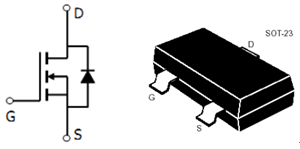SOT-89 封装：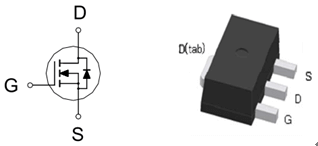### TO 封装

TO（Transistor Out-line，晶体管外形）是比较早期的封装规格，原来多为直插封装（例如 TO-92，TO-220，TO-252），后来也慢慢进化到标贴式封装。TO252 和 TO263 是其典型，其中 TO-252 又称之为 D-PAK，TO-263 又称之为 D2PAK。

D-PAK 封装的 MOS 管有 3 个电极，其中漏极（D）的引脚被剪断不用，而是用背面的散热板作为漏极，能输出更大电流的同时也能更好地散热。

TO-252 封装：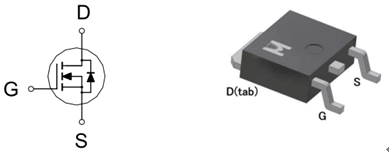TO-263 封装：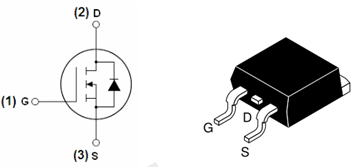### SOP 封装

SOP（Small Out-Line Package，小外形封装），也叫 SO、SOL 或 DFP。通常有 SOP-8、SOP-16、SOP-20、SOP-28 等等（数字表示引脚数）。MOS 的 SOP 封装多数采用 SOP-8 规格。

SOP-8 封装：